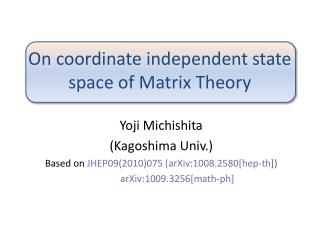# On coordinate independent state space of Matrix Theory - PowerPoint PPT PresentationDownload PresentationOn coordinate independent state space of Matrix Theory

On coordinate independent state space of Matrix TheoryDownload Presentation## On coordinate independent state space of Matrix Theory

- - - - - - - - - - - - - - - - - - - - - - - - - - - E N D - - - - - - - - - - - - - - - - - - - - - - - - - - -
##### Presentation Transcript

1. On coordinate independent state space of Matrix Theory YojiMichishita (Kagoshima Univ.) Based on JHEP09(2010)075 (arXiv:1008.2580[hep-th]) arXiv:1009.3256[math-ph]

2. Introduction Matrix Theory (Banks-Fischler-Shenker-Susskind ‘96) • SU(N) Quantum mechanics ← 10D SYM : bosonic coordinate matrices ( : SO(9) index) : fermionic partners components • N→∞ : 11D M-theory N finite: DLCQ M-theory

3. describes N D0-branes = N KK modes of 1 unit of KK momentum • Multiparticle states → continuous spectrum (de Wit-Lüscher-Nicolai ‘89) • KK modes of 2 or more units realized as bound states → discrete spectrum Conjecture: SU(N) Matrix Theory has a unique normalizable zero energy bound state.

4. Calculation of Witten index seems consisitent. (Yi ‘97, Sethi-Stern ’97, etc.) • Some information: asymptotic form, symmetry (Hoppe et al., etc.) • No explicit expression is known. (for zero energy bound state, and any other gauge invariant wavefunctions)

5. Why is it so difficult? basis of gauge invariant wavefunctions → creation operators → states! (16,777,216 states even for SU(2)) bosonic variables → Schrödinger eq. → equations with variables

6. Enormous number of states and variables → Even numerical calculation is difficult. systematic classification of these states by representation of SU(N)×SO(9) ?

7. Plan • Introduction • Explicit construction of some coordinate independent states in SU(2) case • Number counting of representations in SU(2) case • SU(N) case • Summary

8. Explicit construction of some coord. indep. states in SU(2) case Wavefunction of zero energy bound state • Gauge invariant • SO(9) invariant (Hasler-Hoppe ‘02) • Asymptotic form (SU(2)) Taylor expansion around the origin

9. : coordinate independent states Zero energy → (supercharge ) → ⁞

10. Coord.indep. states for fixed → 256 states : symmetric traceless (44) : antisymmetric (84) : vector-spinor (128) Action of on these states:

11. full states etc. • How do these states transform under gauge transformation? Not immediately clear It is read off by acting generators of the gauge group:

12. SU(2) case • The 1st term (SU(2)×SO(9) singlet) of the expansionhas been constructed and it is unique (up to rescaling). (Hoppe-Lundholm-Trzetrzelewski ’08, Hynek-Trzetrzelewski ‘10)

13. 2nd term ? zero energy → Let us construct coord. Indep. (adjoint)×(vector) representation of SU(2)×SO(9), and see if it satisfies . First let us see the procedure of the construction of representations in a simpler case i.e. singlet case.

14. Decomposition of SO(9) singlets into SU(2) representations • Enumerate SO(9) singlets: 14 states

15. Compute representation matrix of • Eigenvalue spectrum 0, 0, ±1, ±2, ±3, ±4, ±5, ±6 → 1 singlet and 1``spin 6” representation (SU(2) → )

16. Ladder operator Eigenvalue 6 → unique eigenvector ↓ ↓ ⁞ ↓ → orthogonal →

17. Decomposition of SO(9) vectors into SU(2) representations • Enumerate SO(9) vectors: 36 states ⁞

18. Representation matrix of • Eigenvalue spectrum →1 ``spin 1”, 1 ``spin 3”, 1 ``spin 5”, 1 ``spin 7”

19. Spin 1 (adjoint) repr. This is the unique candidate for the 1st order term of the expansion of . Does this satisfy ? YES.

20. Number counting of representations in SU(2) case Explicit construction → too cumbersome Number counting can be done more efficientlyby using characters in group theory. Character for repr.

21. Orthogonality relation Consider the following quantity and decompose it into SU(2)×SO(9) characters: → repr. : multiplicity

22. Computation of the character (Cartansubalgebra part) States:

23. decomposition into SU(2) characters decomposition into SO(9) characters → orthogonality relations

24. SO(9) representations are indicated by Dynkin labels. (72 representations) SU(2) representations are indicated by spins. The unique SU(2)×SO(9) singlet Other states we have constructed. • result This means is automatically satisfied.

25. SU(N) case As in SU(2) case, cannot be separated into creation and annihilation operators without spoiling mainifest SU(N)×SO(9) symmetry. Several different ways of respecting symmetries

26. SU(3) case ( =18,446,744,073,709,551,616 states) Decomposing into SU(3) characters first: ⁞ 1454 singlets!

27. Decomposing into SO(9) characters first: ⁞ 1454 singlets!

28. Many SU(3)×SO(9) singlets → does not mean that there are many bound states. (The power series may give nonnormalizable states, or and other equations may not have nontrivial solution.) • SU(4), SU(5), SU(6), ….. ???

29. Summary • In SU(2) case we explicitly constructed some coord. Indep. states in lower repr. of SU(2)×SO(9) →give lower terms in Taylor expansion of zero energy normalizablewavefunction. • In SU(2) case we counted the multiplicity of representations of SU(2)×SO(9) by computing the character.

30. In SU(N) case we computed the character and saw some multiplicities. →many singlets • Exact expression of zero energy bound state or other states? • Application to scattering or decay process?